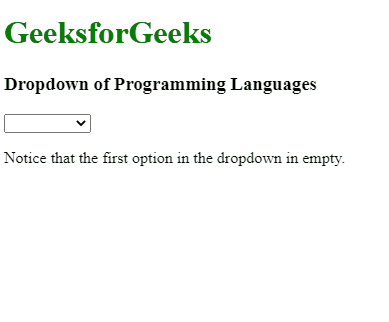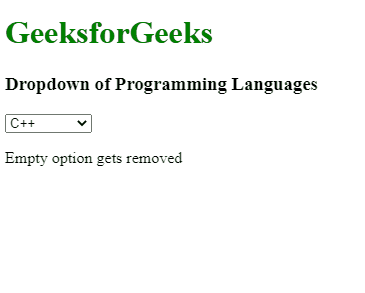Open In App

# Why does AngularJS include an empty option in select ?

AngularJS is a Javascript framework containing several built-in functionalities, that intend to make the development easier and more efficient for the developers. The dropdown is one of them. A dropdown is a GUI element that enables the user to choose one value from a list of values. When a drop-down is active, a list of values gets displayed to the user, out of which one value can be selected at a time. After a value is selected, the drop-down gets back to its default state. However, while working with the AngularJS dropdown, it was observed that the first option in the select dropdown is always empty. Have a look at the following example for a better understanding.

Example 1: The below example illustrates an empty option in the select menu in AngularJS.

## HTML

 ```<``html``>`` ` `<``head``>``    ``<``script` `src``=``"https://cdnjs.cloudflare.com/ajax/libs/angular.js/1.0.3/angular.js"` `            ``integrity``=``"sha256-ZYJ4XH6kZrqD1MlZWZZmQj6MBe/TvysMc33LPchVuo8="` `            ``crossorigin``=``"anonymous"``>``    `````` ` `<``body``>``    ``<``h1` `style``=``"color:green;"``>``        ``GeeksforGeeks``    ````    ``<``h3``>``        ``Dropdown of Programming Languages``    ````    ``<``div` `ng-App``=``"myApp"` `ng-controller``=``"MyCtrl"``>``        ``<``select` `ng-model``=``'form.type'``             ``ng-options``=``'name.val as name.label for name in allNames'``>``        ````    ````    ``<``p``> Notice that the first option in the dropdown in empty. ``    ``<``script``>``        ``var myApp = angular.module('myApp', []);``        ``function MyCtrl(\$scope) {``            ``\$scope.allNames = [{``                ``label: 'C',``                ``val: 'C Language'``            ``}, {``                ``label: 'C++',``                ``val: 'C++ Language'``            ``}, {``                ``label: 'Java',``                ``val: 'Java Language'``            ``}, {``                ``label: 'Python',``                ``val: 'Python Language'``            ``}, {``                ``label: 'JavaScript',``                ``val: 'JavaScript Language'``            ``}];``        ``}``    `````` ` ``

Output:Explanation and Correction: Generally, a list of options are transferred to ng-option directive. Those options are then displayed to the user in GUI format. However, when some value referenced by the ng-model directive doesn’t exist in the list transferred to the ng-option, then an empty option gets created in the GUI list, which can be seen above example. The easiest way to correct this error is to reference a valid value from the ng-option list. The value referenced must be available on the list. We can reference a valid value using the below code. Here, i will be the index of the value that we want to reference.

`\$scope.form = {type : \$scope.allNames[i].val}; `

Just add this code below the list to remove the empty option from the select. In the example given below, we are modifying the above example, in such a way that the empty option gets removed from the drop-down. We will set the value of i to 1. After doing this, the empty option will disappear and the default selected value will be set to C++.

Example 2: This example illustrates removing the empty option by selecting an initial value in the controller in AngularJS.

## HTML

 ```<``html``>`` ` `<``head``>``    ``<``script` `src``=``"https://cdnjs.cloudflare.com/ajax/libs/angular.js/1.0.3/angular.js"``            ``integrity``=``"sha256-ZYJ4XH6kZrqD1MlZWZZmQj6MBe/TvysMc33LPchVuo8="` `            ``crossorigin``=``"anonymous"``>``    `````` ` `<``body``>``    ``<``h1` `style``=``"color:green;"``>``        ``GeeksforGeeks``    ````    ``<``h3``>``        ``Dropdown of Programming Languages``    ````    ``<``div` `ng-App``=``"myApp"``         ``ng-controller``=``"MyCtrl"``>``        ``<``select` `ng-model``=``'form.type'` `             ``ng-options``=``'name.val as name.label for name in allNames'``>``        ````    ````    ``<``p``>Empty option gets removed``    ``<``script``>``        ``var myApp = angular.module('myApp', []);``     ` `        ``function MyCtrl(\$scope) {``            ``\$scope.allNames = [{``                ``label: 'C',``                ``val: 'C Language'``            ``}, {``                ``label: 'C++',``                ``val: 'C++ Language'``            ``}, {``                ``label: 'Java',``                ``val: 'Java Language'``            ``}, {``                ``label: 'Python',``                ``val: 'Python Language'``            ``}, {``                ``label: 'JavaScript',``                ``val: 'JavaScript Language'``            ``}];``            ``\$scope.form = {``                ``type: \$scope.allNames.val``            ``};``        ``}``    ```````

Output: